#### Andrew Chap

*Auto-zoom is disabled because it doesn't work well with Plotly. Please set your browser to full-screen and zoom in or out until the slides take up the entire page.

# INTRODUCTION

### The 1-D Child-Langmuir Law

Two boundaries:

• Separated by distance $d$ (domain length)
• Voltage bias $V$
• Particles injected at left boundary with $v_0 = 0$

$J_\mathrm{SCL}$ is the maximum current that can cross the domain due to Space-Charge-Limiting:

$$J_\mathrm{SCL} = \frac{4}{9}\epsilon_0\sqrt{\frac{2 e}{m}}\frac{V^\frac{3}{2}}{d^2}$$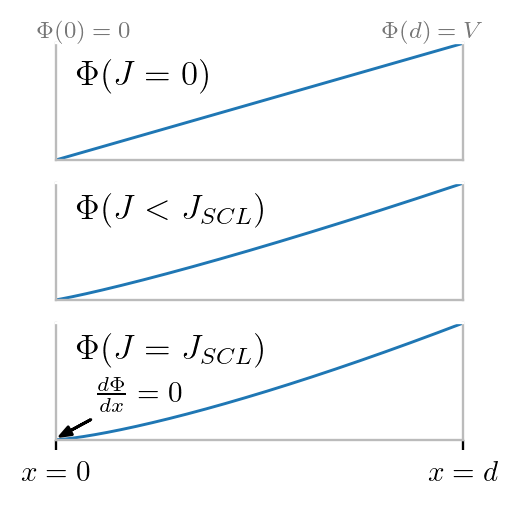### $\Phi$, $E$, $v$, and $\rho$

Generalize: $d\rightarrow x$, $V \rightarrow \Phi(x)$

$\color{blue}{\Phi(x)} = \left(\frac{9}{4} \frac{J_\mathrm{SCL}}{\epsilon_0}\right)^{\frac{2}{3}} \left(\frac{m}{2e} \right)^{\frac{1}{3}} \color{blue}{x^\frac{4}{3}}$

$\color{green}{E(x)} = \frac{d\color{blue}{\Phi(x)}}{dx} = \frac{4}{3}\left(\frac{9}{4} \frac{J_\mathrm{SCL}}{\epsilon_0}\right)^{\frac{2}{3}} \left(\frac{m}{2e} \right)^{\frac{1}{3}} \color{green}{x^\frac{1}{3}}$

$\color{purple}{v(x)} = \sqrt{\frac{2 e\color{blue}{\Phi(x)}}{m}} = \left(\frac{9}{2} \frac{e J_\mathrm{SCL}}{m\epsilon_0}\right)^{\frac{1}{3}}\color{purple}{x^\frac{2}{3}}$

$\color{red}{\rho(x)} = \frac{J_\mathrm{SCL}}{\color{purple}{v(x)}} = \left(\frac{2}{9} \frac{m \epsilon_0 J^2_\mathrm{SCL}}{e}\right)^{\frac{1}{3}}\color{red}{x^{-\frac{2}{3}}}$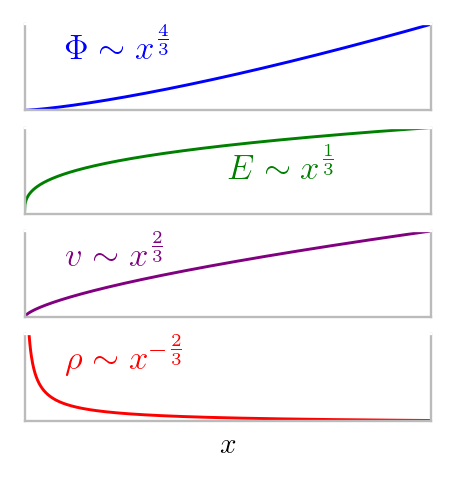### Simulating Space-Charge-Limited (SCL) Emission in 1D

Because the equations for SCL flow are self-similar, we can simulate SCL emission on the left boundary without any information from the right boundary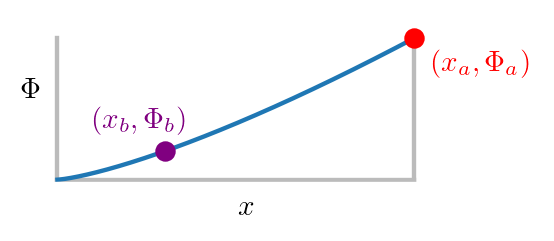$$\color{red}{J_\mathrm{SCL}\left(x_a,\Phi_a\right)} = \color{purple}{J_\mathrm{SCL}\left(x_b,\Phi_b\right)}$$Plasma,

Complex Geometry

etc...

$$\color{purple}{\left(\Delta x,\Phi(\Delta x)\right)}$$

To simulate the local emission at the left boundary, we can calculate

$$J_\mathrm{emit} = J_\mathrm{SCL}(\Delta x, \Phi(\Delta x))$$

We can use $\Phi(\Delta x) = E_0\Delta x$ so that our emitted current is:

$$J_\mathrm{emit} = \frac{4}{9}\epsilon_0\sqrt{\frac{2 e}{m}}\frac{E_0^\frac{3}{2}}{\sqrt{\Delta x}}$$

where $E_0 = E(x=0)$ is the surface electric field and we take $\Phi(x=0)=0$.

### Simulating Space-Charge-Limited (SCL) Emission in 1D

So we have: $J_\mathrm{emit} \sim \dfrac{E_0^\frac{3}{2}}{\sqrt{\Delta x}}$

Looks strange... because

• In space-charge-limited emission, $E_0$ should be zero

• Our emitted current goes as $\Delta x^{-\frac{1}{2}}$

But...

• Because of finite differencing, we will never get $E_0 = 0$, because $E_0 = \frac{\Phi(\Delta x) - \Phi(0)}{\Delta x}$ with $\Phi \sim x^\frac{4}{3}$
• Since $E \sim x^\frac{1}{3}$, we have $E^\frac{3}{2} x^{-\frac{1}{2}} \sim 1$

# ELECTROSTATICS

### Results: $J_\mathrm{SCL}$ vs spatial resolution

Test Problem:

$V = 1\,\mathrm{Volt}$, $L = 1\,\mathrm{meter}$

Electrons emitted from left boundary

Steady-state results from calculating $J_\mathrm{SCL}$ from the self-consistent electric field at the left surface

$$\mathrm{Error}\left[J_\mathrm{SCL}\right]\sim \Delta x^\frac{2}{3}$$

### Results: $J_\mathrm{SCL}$ vs spatial resolution

Test Problem:

$V = 1\,\mathrm{Volt}$, $L = 1\,\mathrm{meter}$

Electrons emitted from left boundary

Steady-state results from calculating $J_\mathrm{SCL}$ from the self-consistent electric field at the left surface

$$\mathrm{Error}\left[J_\mathrm{SCL}\right]\sim \Delta x^\frac{2}{3}$$

### Results: $J_\mathrm{SCL}$ vs spatial resolution

Test Problem:

$V = 1\,\mathrm{Volt}$, $L = 1\,\mathrm{meter}$

Electrons emitted from left boundary

Steady-state results from calculating $J_\mathrm{SCL}$ from the self-consistent electric field at the left surface

$$\mathrm{Error}\left[J_\mathrm{SCL}\right]\sim \Delta x^\frac{2}{3}$$

Same test as before except electrons reflected at right boundary

Theoretical result: $\left[J_\mathrm{SCL}\right]_\mathrm{two-way} = \frac{1}{2} \left[J_\mathrm{SCL}\right]_\mathrm{one-way}$ since reflected particles create twice the space charge

• In numerical experiments, $\left[J_\mathrm{SCL}\right]_\mathrm{two-way} \lt \frac{1}{2} \left[J_\mathrm{SCL}\right]_\mathrm{one-way}$ because not all particles have exactly the energy needed to return to the cathode, some get trapped and create a virtual cathode that reduces $J_\mathrm{SCL}$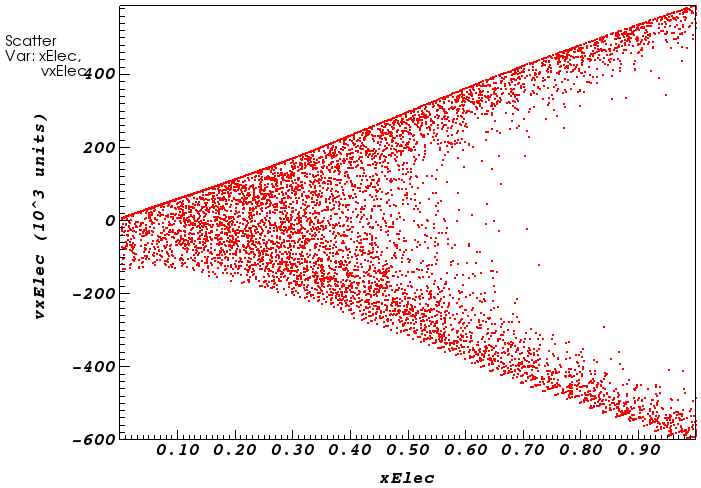$J_\mathrm{SCL}(t)$

$N_\mathrm{electrons}(t)$

$\Phi(x)$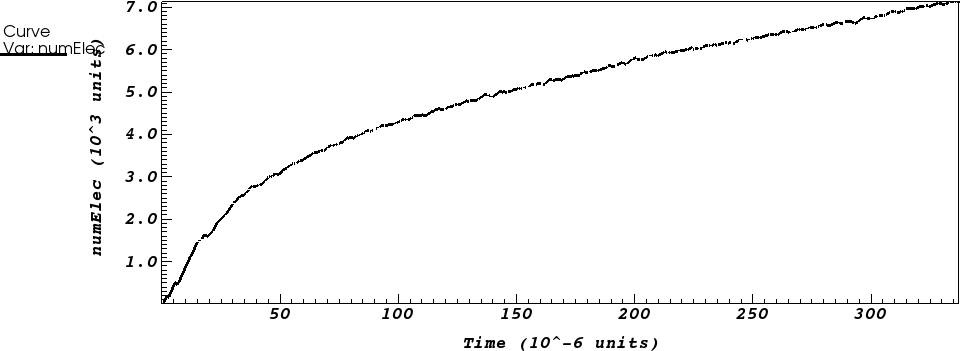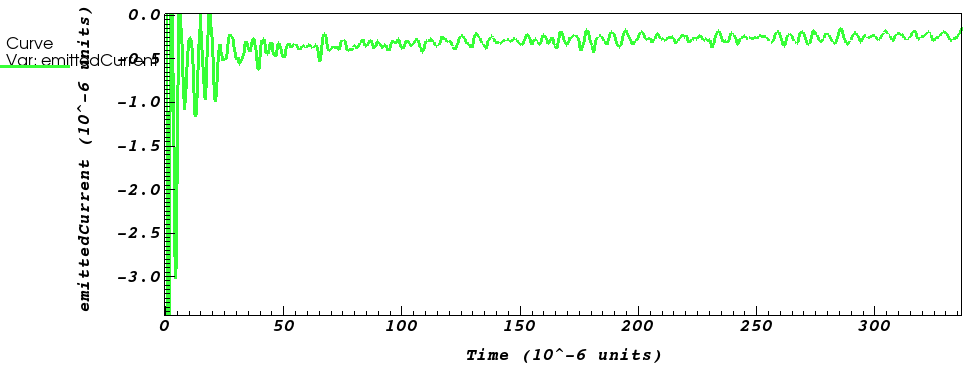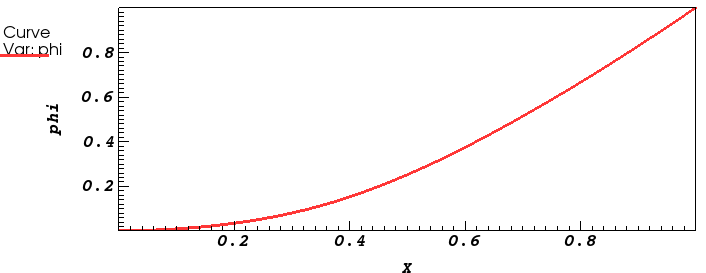# ELECTROMAGNETICS

### 3D SCL EM parallel plates

Issues with electromagnetic SCL

• Can't specify voltage in EM!
• $\frac{1}{c^2}\frac{d\mathbf{E}}{dt} = \mu_0\mathbf{J}$: Constant $J_\mathrm{SCL}$ means $E$ keeps increasing! Results in oscillatory solution, "virtual cathode"

Workaround: impose displacement current density $J_\mathrm{D}$, then in steady-state: $J_\mathrm{SCL} = J_\mathrm{D}$

Integrate $E$ across the gap to find $V$ across the gap, compare to $V_\mathrm{SCL} = \left(\frac{9}{4} \frac{J_\mathrm{D}}{\epsilon_0}\right)^{\frac{2}{3}} \left(\frac{m}{2e} \right)^{\frac{1}{3}} L^\frac{4}{3}$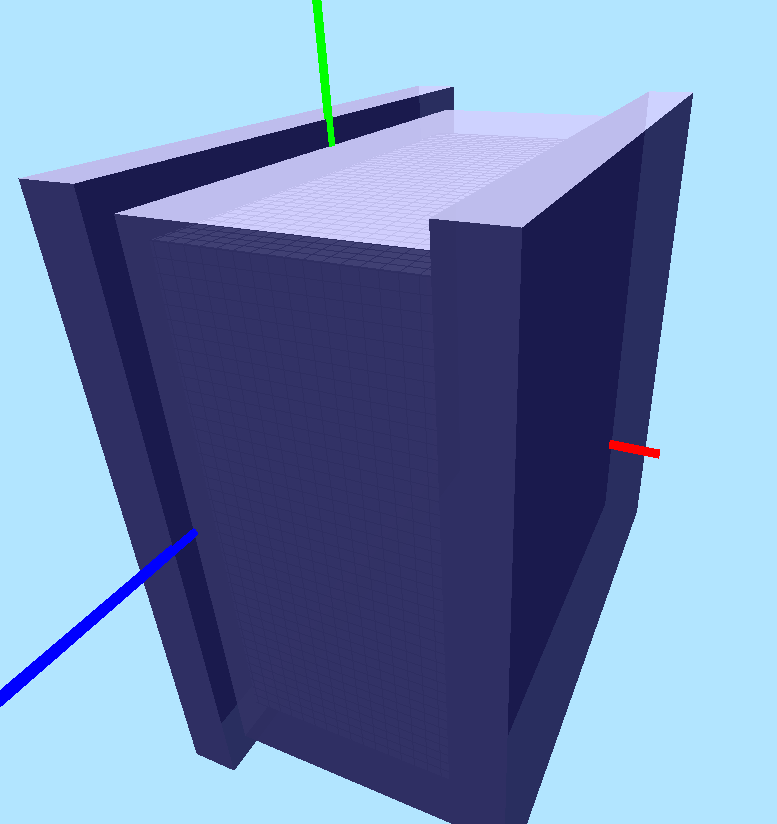Impose $J_{\mathrm{D},x}$ periodic boundaries in $y$ and $z$

### 3D SCL EM parallel plates continued

Test Problem: $J_\mathrm{D} = 250\,\frac{\mathrm{A}}{\mathrm{m}^2}$, $L = 10\,\mathrm{cm}$

$V_\mathrm{SCL} = \left(\frac{9}{4} \frac{J_\mathrm{D}}{\epsilon_0}\right)^{\frac{2}{3}} \left(\frac{m}{2e} \right)^{\frac{1}{3}} L^\frac{4}{3} = 10.5\,\mathrm{kV}$

Baseline simulation: funcVelGen with $J_\mathrm{emit} = 1.25J_\mathrm{D}$

$\left[V_\mathrm{SCL}\right]_\mathrm{funcVelGen} = 11.3\,\mathrm{kV}$

Test: childLangmuirVelGen with no emit current specified

$\left[V_\mathrm{SCL}\right]_\mathrm{childLangmuirVelGen} = 10.9\,\mathrm{kV}$

$\left[\color{black}{V_\mathrm{SCL}}\right]_\mathrm{funcVelGen}$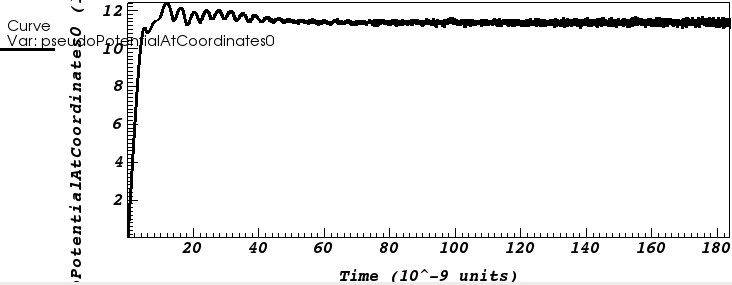$\left[\color{black}{V_\mathrm{SCL}}\right]_\mathrm{childLangmuirVelGen}$### 3D SCL EM parallel plates continued

Test Problem: $J_\mathrm{D} = 250\,\frac{\mathrm{A}}{\mathrm{m}^2}$, $L = 10\,\mathrm{cm}$, $A = 0.04\,\mathrm{m}^2$, $J_D = 10 \,\mathrm{A}$

$\left[\color{green}{J_\mathrm{cathode-emit}},\color{blue}{J_\mathrm{cathode-absorb}},\color{red}{J_\mathrm{anode-absorb}}\right]_\mathrm{funcVelGen}$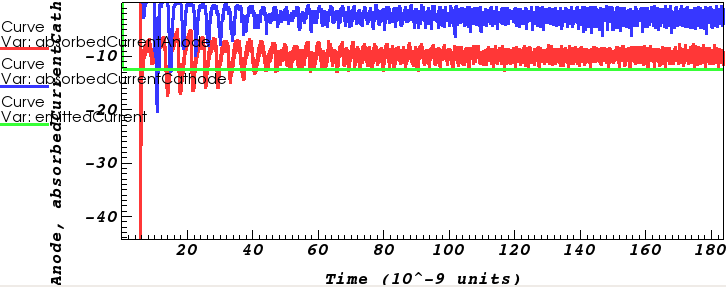$\left[\color{green}{J_\mathrm{cathode-emit}},\color{blue}{J_\mathrm{cathode-absorb}},\color{red}{J_\mathrm{anode-absorb}}\right]_\mathrm{childLangmuirVelGen}$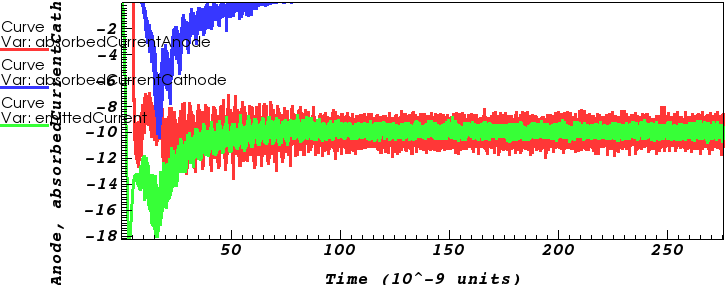### Testing SCL cut-cell emission

Take David Smithe's example, and rotate the plates so that they are not grid-aligned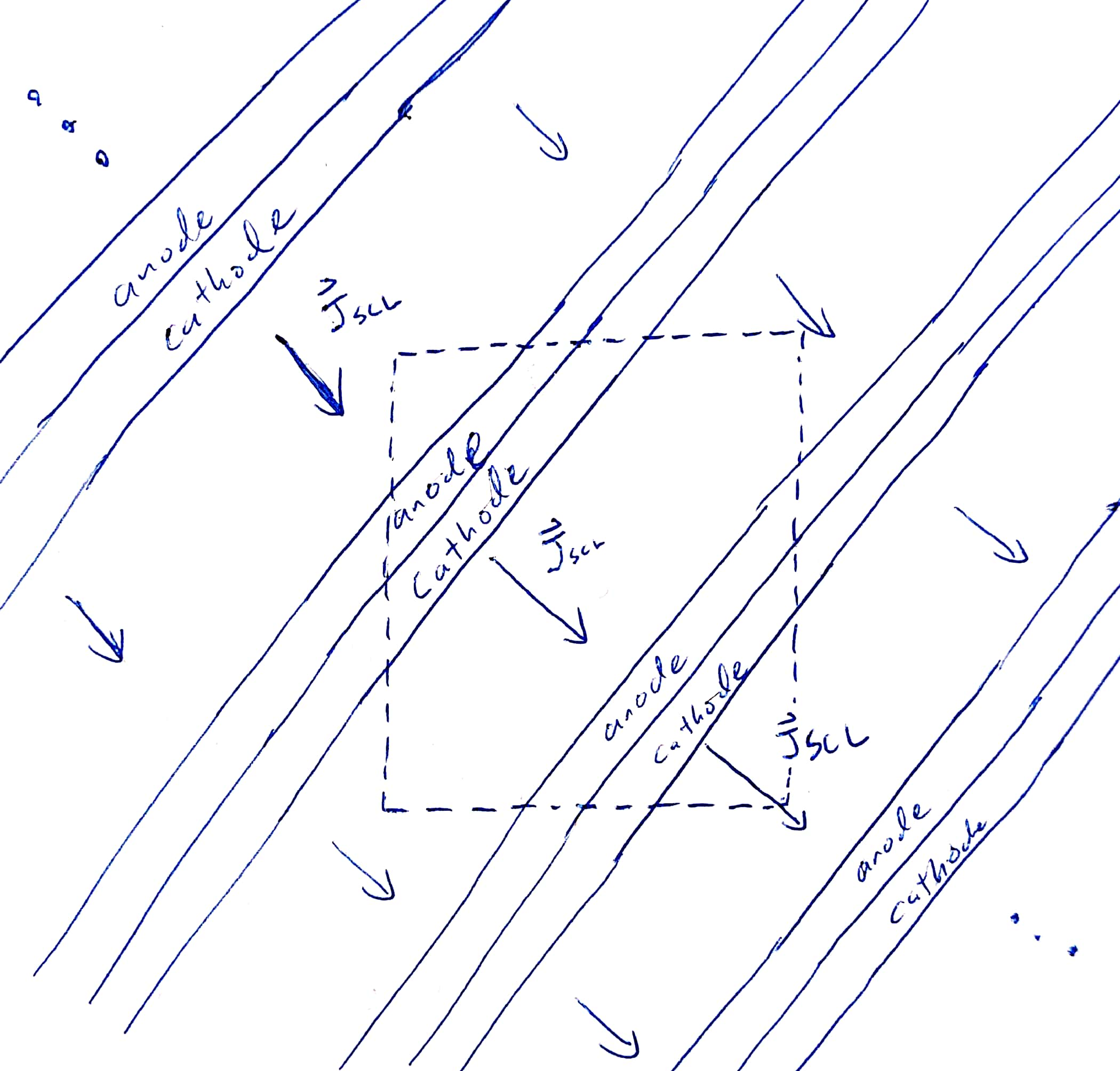Periodic boundaries in $x$, $y$, and $z$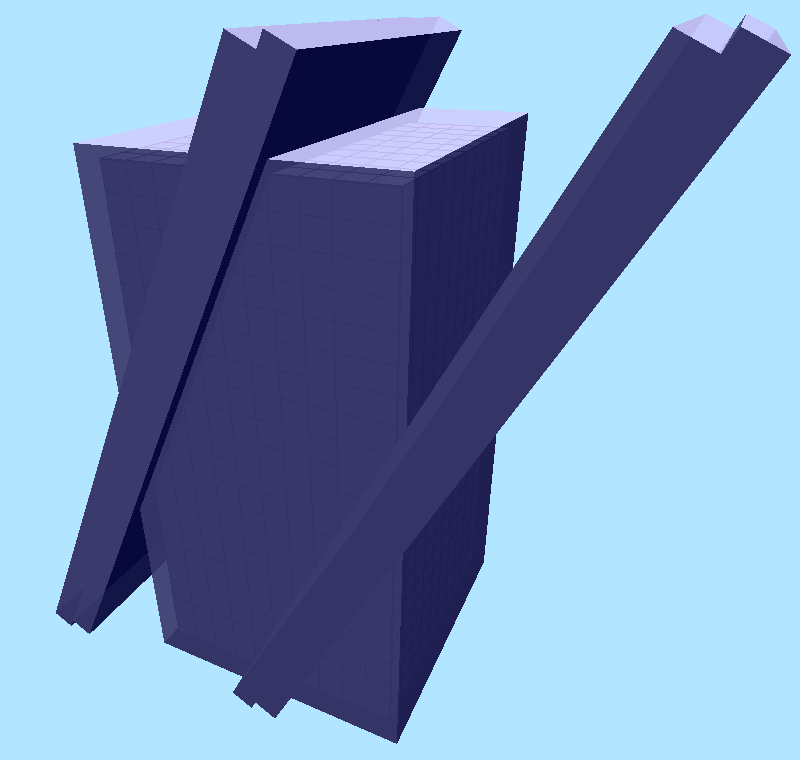Electrodes intersect boundaries such that they are periodic as well

### Testing SCL cut-cell emission

Found strange electron "banding" in both funcVelGen & childLangmuirVelGen

Since $E_\mathrm{normal} \rightarrow 0$, even a small amount of $E_\parallel$ will cause electrons to move parallel to the emitting surface.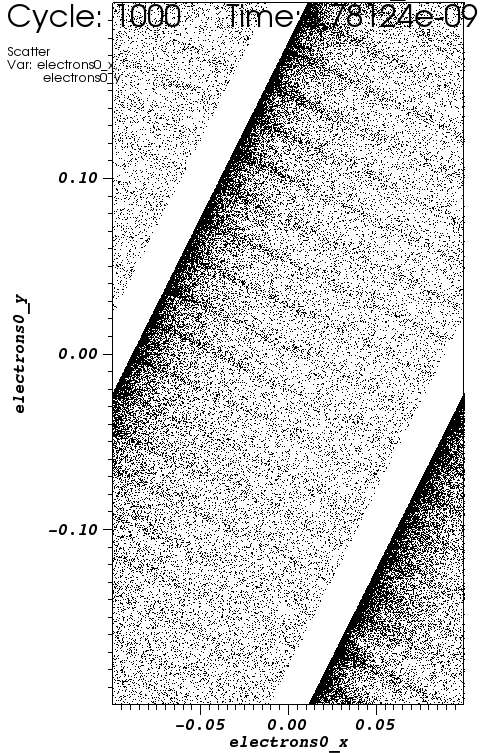# TEMPORALRESPONSE

### Slow Voltage Rise

Voltage rises over a few crossing times

$$\downarrow$$

### Fast Voltage Rise

Voltage rises over less than a crossing time

$$\downarrow$$

$J_\mathrm{emit}$ oscillates

# APPLICATIONS

### Magnetically Insulated Line Oscillator (MILO)

Pulsed power microwave device
DC power → electron current → RF power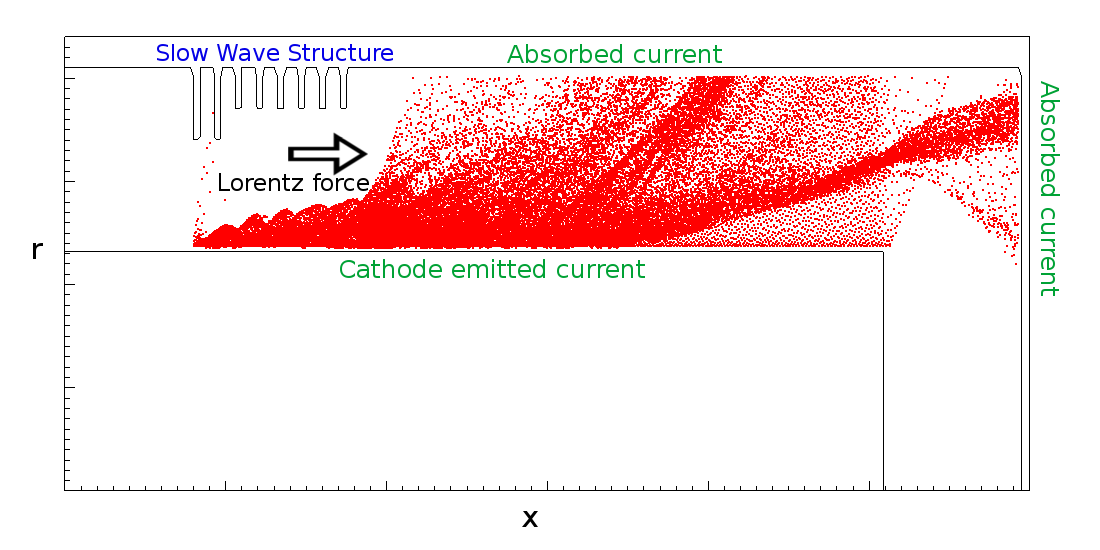##### Grid Resolution Depencence:

Slow wave structure at twice the resolution: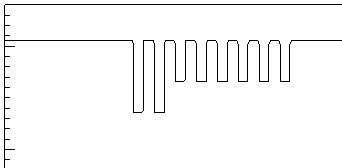Slow wave structure at half the resolution: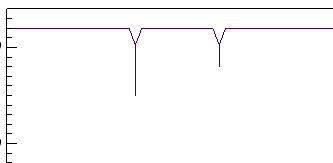### MagnetronCathodeSimulationwith theSCL Emitter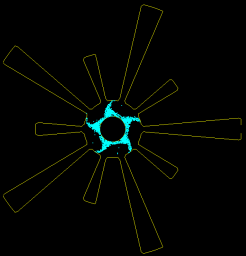### MagnetronCathodeSimulationwith theConstant current source# FUTURE WORK

### Future work

• Currently working on $v_0>0$ that follows $$J_\mathrm{SCL}(v \gt 0) = J_\mathrm{SCL}(v=0)\left[\left(1 + \frac{\frac{1}{2}mv^2}{eV}\right)^\frac{1}{2}\right]^3$$

Thermal emitter?

Requires numerical solution to find maximum $J_\mathrm{SCL}$ (excluding return current) for $J_\mathrm{SCL}\left(L\mathrm{gap},V_\mathrm{gap},T_\mathrm{surface}\right)$

• Option to make the particle weights more consistent, by specifying macroparticle weight and maximum particles emitted per cell in a step